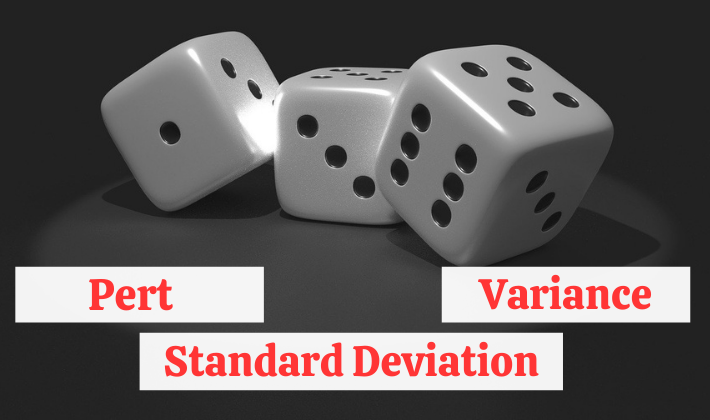## Three Point Estimate Formulas

Quick Reference on Three Point Estimate I have written a series of articles on Three Point Estimate and related concepts. Before reading this, you should look at my first article of the series. I have explained the basic concept of Three Point Estimate in my first article. I have also explained that PERT is not…## Did You Know That A project Is Scheduled To FAIL?

A project is scheduled to FAIL from start. This is a very strong statement albeit true. I have noticed this phenomenon many times during my experience. Let me explain. Project Managers rarely understand good scheduling concepts/techniques. Even if they understand, good concepts/techniques are rarely used while developing project schedule. What are good scheduling concepts/techniques? These…## Variance And Standard Deviation In PERT Formula [PMP]

You might be looking to understand the usage of variance and standard deviation in PERT formula. PERT is an acronym for Program Evaluation and Review technique. This technique is used to determine duration and cost estimates of a task. In this technique, a weighted average (mean) of three different estimates is used to determine the…## How To Use PERT Formula For Three Point Estimating?

PERT Formula is one on the ways for doing three point estimation. It calculates expected duration by finding the weighted average of three different estimates viz. Optimistic (O), Pessimistic (P), and Most Likely (M). E_PERT=(O+P+4×M)/6 The three point estimation can also be done by using simple average formula. E_SA=(O+P+M)/3 PERT (Program Evaluation and Review Technique)…